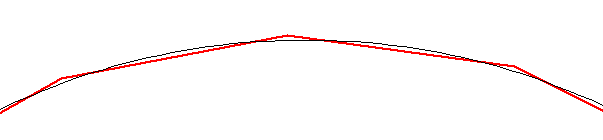Index Previous Next

## AspEdit manual      Circles

There are two types of circles:
• Full circles.  They require:
• The center point,

• Circle segments. They require:
• The start point.  This is the last point in the area before the circle segment
• The center point,
• The end point
• The sense of rotation.
Notes:
• For circle segments, the radius is calculated from the distance of the start & end points from the center.
• It is possible to define a full circle using the same start & end point and a center point.  Use this if the circle is running through a given point and the radius is not given.

### Inserting a circle / circle segment

• Via the "Block" dialog box
• Copy the entire shape into the input field of the dialog box. circle (segments) will be recognised automatically
• Leave the block dialog box and check the result on the map

• Via the "Add / Edit Circle" dialog box
1. For circle segments (not required for full circles), make sure that at least one point is defined before the segment.  The last point before the segment will be the starting point.
2. In the Area dialog box, press the add or insert button.
3. The Add element dialog boxwill open.
4. Select "Circle (or segment)", the Circle dialog box will open.
5. Enter the fields as required.

### Modifying a circle segment

In the list of the Area dialog box, double-click on any line belonging to the circle (segment), the Circle dialog box will open for the selected segment

### Drawing quality of circles on the map

Via the Map settings dialog box, you can select the drawing quality of circles.  The options are: low / medium / high.
In either case, the points are calculated such that the deviation from the exact circle is minimized, see the picture below.The black line is the exact circle, the red line is the circle along the calculated points.

### Quality of exported circles

When no shapes were added or subtracted to the shape, circles are exported into OpenAir format using the DC and DB records.  It is up to the target device to expand the circles to the adequate quality.
When shapes were added or subtracted, all features in it will be expanded.  For circles, the same resolution as selected for the map will be used.  This allows to trade resolution for the number of points.